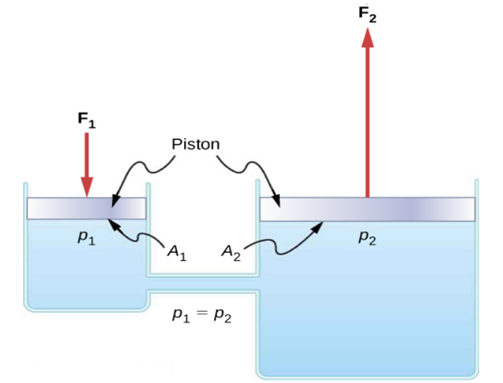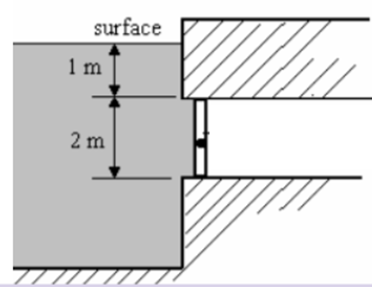We're Open

100% Pass and No Plagiarism Guaranteed

# 1.1 Describe the molecular and physical properties of fluids

Fluid mechanics

Understand the fundamental principles of fluids

 1.1 Describe the molecular and physical properties of fluids Define what is meant by a fluid. Briefly explain the molecular structure in fluids. Explain why a drop of liquid will take a spherical shape in the absence of a container. 1.2 Explain the application of Pascal’s Law to engineering systems and carry out associated calculations Consider the following hydraulic system:Explain Pascal’s Law and use it to explain what effect increasing the pressure at point 1 has on point 2 in the hydraulic system. A car repair shop uses a lift to raise a 160kg engine. The lift has a large piston with a diameter of 24cm. To raise the lift, force is exerted on a small piston with a diameter of 8cm. What force must be exerted to raise the lift? What is the mechanical advantage of the hydraulic system? The smaller piston is pushed down by 1.5m, how much does this raise the larger piston? 1.1 Describe the molecular and physical properties of fluids 1.3 Explain and apply Archimedes Principle to engineering problems. Explain Archimedes’ principle. Explain why objects with a specific gravity of less than one will float in water. A spherical buoy of diameter 0.3 m and mass 4.2 kg is attached to the seabed by a mooring rope and floats fully submerged. Calculate the tension in the mooring rope. The density of the seawater is 1025 .
 MERIT 1.4 Calculate forces and centre of pressure on submerged surfaces.Consider the underwater hatch in the scenario pictured below: The hatch is rectangular and has a height of h = 2m and a width of b = 3m and does not run to the surface. Find the force needed to be applied at the top of the hatch to keep it closed. The fluid the hatch is submerged in is water with density 1020 DISTINCTION 1.5 Discuss the structure of the atmosphere and the change in parameters with altitude. Describe the different layers which exist within the atmosphere. Describe in detail how the following properties of air change with altitude: Density Temperature Pressure

Un derstand the Effects of Viscosity in Real Fluids

 2.1 Explain the characteristics of and parameters of viscosity in fluids. Explain what is meant by the no slip condition. Explain the difference between dynamic and kinematic viscosity. Calculate the kinematic viscosity for a fluid that has a dynamic viscosity of  and density of 1000.  Calculate the kinematic viscosity for a fluid that has a dynamic viscosity of 8.9×10〖^(-4)〗kgm〖^(-1)〗s〖^(-1)〗 and density of 1000kgm〖^(-3)〗. 2.2 Describe viscosity measurement techniques Describe the way in which viscosity can be measured using: An Ostwald viscometer A Redwood viscometer and A falling sphere viscometer The viscosity of an oil is to be measured using the falling sphere method. The oil has a density of 850 the sphere has a density of 1145 and a diameter of 4.5mm. The sphere is dropped into the fluid, it reaches a terminal velocity of 6mm/s. The correction factor for this experiment is 1.9 (the amount the viscous drag increases due to the diameter of the cylinder being low and interfering with the sphere) 1.1 Describe the molecular and physical properties of fluids The viscosity of an oil is to be measured using the falling sphere method. The oil has a density of 850kgm〖^(-3)〗, the sphere has a density of 1145kgm〖^(-3)〗 and a diameter of 4.5mm. The sphere is dropped into the fluid, it reaches a terminal velocity of 6mm/s. The correction factor for this experiment is 1.9 (the amount the viscous drag increases due to the diameter of the cylinder being low and interfering with the sphere) Determine the dynamic and kinematic viscosity of the oil.
 2.3 Describe the effects of temperature and shear forces on Newtonian and non-Newtonian fluids. Describe and explain the effect of increasing temperature on the viscosity of liquids and gases. Describe what is meant by shear rate within a fluid. Explain the effect on viscosity when the shear rate is increased for Newtonian and non-Newtonian fluids. 2.4 Apply dimensional analysis to fluid flow problems. A ball is in a stationary condition in flowing ideal liquid, the velocity of which is V and density ρ. The radius of the ball is R. Find out an expression for dynamic pressure, P, on the ball and indicate whether this is dependent on the radius in the final expression. You may initially assume that the pressure on the ball is related to the density, radius and velocity so that: P=constant×ρ〖^a〗V〖^b〗R〖^c]

Evaluate Aerodynamic Forces in Fluid Flow

Evaluate Aerodynamic Forces in Fluid Flow

 3.1 Apply continuity and Bernoulli equations to incompressible fluid problems Water is flowing through a tapering duct below: The diameter of the duct decreases from 400mm to 300mm. The velocity at the larger section of the duct is 6m/s. Calculate: The velocity at the smaller diameter. The volume flow rate. The mass flow rate. You may assume the density of the water is 1000. Consider the hose below: The pressure of the water at point 2 is 112kPa. Find the velocity and the pressure of the water at point 1. You may assume the density of the water is 1000. 3.2 Define and calculate Reynolds number and relate this to the physical properties of the flow Consider a fluid flowing in a pipe. Describe how increasing each of the quantities would affect Reynolds number. Diameter of pipe Flow velocity Density of fluid Dynamic viscosity of fluid Describe the physical properties of the fluid flow at lower Reynolds numbers (below 2300) and explain how the characteristics of the flow would change if the velocity increased and the Reynolds number increased to 5000. Oil is flowing in a pipe, the density of the oil is 860The dynamic viscosity is 0.28 Pa/s, the pipe diameter is 0.06m and the flow velocity is 35m/s. Is this laminar, transitional or turbulent flow? 3.3 Calculate lift and drag forces and coefficients Consider an aircraft wing the has an area of 500. The aircraft is travelling at 200m/s through air that has a density of 0.8. The lift coefficient of the wing is 0.65. Calculate the lift force generated under these circumstances. A model wing  is placed in a wind tunnel. The velocity of air in the tunnel is 50m/s with a density of  1.2. The drag force generated is 6kN over an area of 7. Calculate the drag coefficient of the wing.

 3.4 Explain the properties and effects of boundary layers in a flow field. Consider the scenario where fluid is flowing over a surface. The boundary layer develops from laminar to turbulent. Produce a sketch that indicates the following key properties within the boundary layer: Laminar and turbulent boundary layer Transition point Velocity gradient Boundary layer thickness Free stream velocity Explain why fluids which have higher viscosity have a larger boundary layer thickness. Flow can separate when an adverse pressure gradient occurs over the wing of an aircraft. Explain what is meant by an adverse pressure gradient and how this leads to flow separation. Briefly explain the effects of flow separation on the wing. 3.5 Relate properties of fluids to the drag force on bodies in flow fields. Explain what is meant by skin friction drag and explain how this can be reduced for a body. Explain what is meant by pressure drag. Explain what interference drag is and suggest a way in which it could be measured. Describe how lift induced drag is generated. Calculate the induced drag experienced by an aircraft that is in level flight (flying at constant altitude) If the mass of the aircraft is 250000kg, the wingspan is 60m, the density of air at the cruising altitude is 0.7and the true airspeed of the aircraft is 210m/s.

Understand the operating principles of hydraulic machines

Hh

 4.1 Identify and explain the operating principles of water turbines and pumps. Produce a short report that describes the key features and explains the principles of operation for a Pelton turbine, Francis turbine and Kaplan turbine. Explain the key differences between radial flow, axial flow and mixed flow in pumps. Explain what is meant by the pressure head of a pump and calculate the pressure head for a pump that has a useful power of 200W, delivering a flow rate of 0.002for water of density 1000 Explain what is meant by the pressure head of a pump and calculate the pressure head for a pump that has a useful power of 200W, delivering a flow rate of 0.002m〖^3〗/sfor water of density 1000kgm〖^(-3)〗 4.2 Evaluate the impact of a jet of fluid on a stationary plate. A jet of water strikes a plate that is vertically positioned in front of the jet. The density of the water is 1025and the velocity of the jet is 45m/s. The jet emerges from a nozzle with a diameter 2.5cm. Find the force required to hold the plate in position. A jet of water strikes a plate that is vertically positioned in front of the jet. The density of the water is 1025kgm〖^(-3)〗and the velocity of the jet is 45m/s. The jet emerges from a nozzle with a diameter 2.5cm. Find the force required to hold the plate in position. The plate is then inclined 45 degrees, what force is now required
 4.3 Evaluate performance parameters of a hydraulic turbine. A Pelton wheel is supplied with a jet velocity 1.6 times greater than the bucket velocity. The buckets rotate with diameter 2.2m at 1100 rev/min. The water is deflected 165 degrees. Blade friction coefficient, k, is 0.96. Determine the mass flow rate of water needed to develop a shaft power of 2.5MW. The mechanical efficiency of the device is 78%. 4.4 Review and compare flow meters and justify their selection in different applications. Briefly describe how the following flow meters operate: Orifice plate Venturi flow meter Pitot tube Axial turbine flow meter  Suggest which flow meter would be best used in the following applications: Measuring flow rate in a pipe with a larger flow rate and minimal straight sections. A high pressure and temperature flow in a pipe with solid particulates. 1.1 Describe the molecular and physical properties of fluids A venturi meter has a larger cross sectional area of 0.045and a smaller cross sectional area of  0.0018. The differential pressure across the venturi tube is calculated at 11kPa. The density of water flowing through the tube is 1000and the discharge coefficient is 0.87. Calculate the volume flow rate. A venturi meter has a larger cross sectional area of 0.045m〖^2〗and a smaller cross sectional area of 0.0018m〖^2]. The differential pressure across the venturi tube is calculated at 11kPa. The density of water flowing through the tube is 1000kgm〖^(-3) and the discharge coefficient is 0.87. Calculate the volume flow rate

100% Plagiarism Free & Custom Written,International House, 12 Constance Street, London, United Kingdom,
E16 2DQ

## STILL NOT CONVINCED?

We've produced some samples of what you can expect from our Academic Writing Service - these are created by our writers to show you the kind of high-quality work you'll receive. Take a look for yourself!